Whole Number Exponents Math Video for Kids - 6th, 7th, & 8th Grades
1%
It was processed successfully!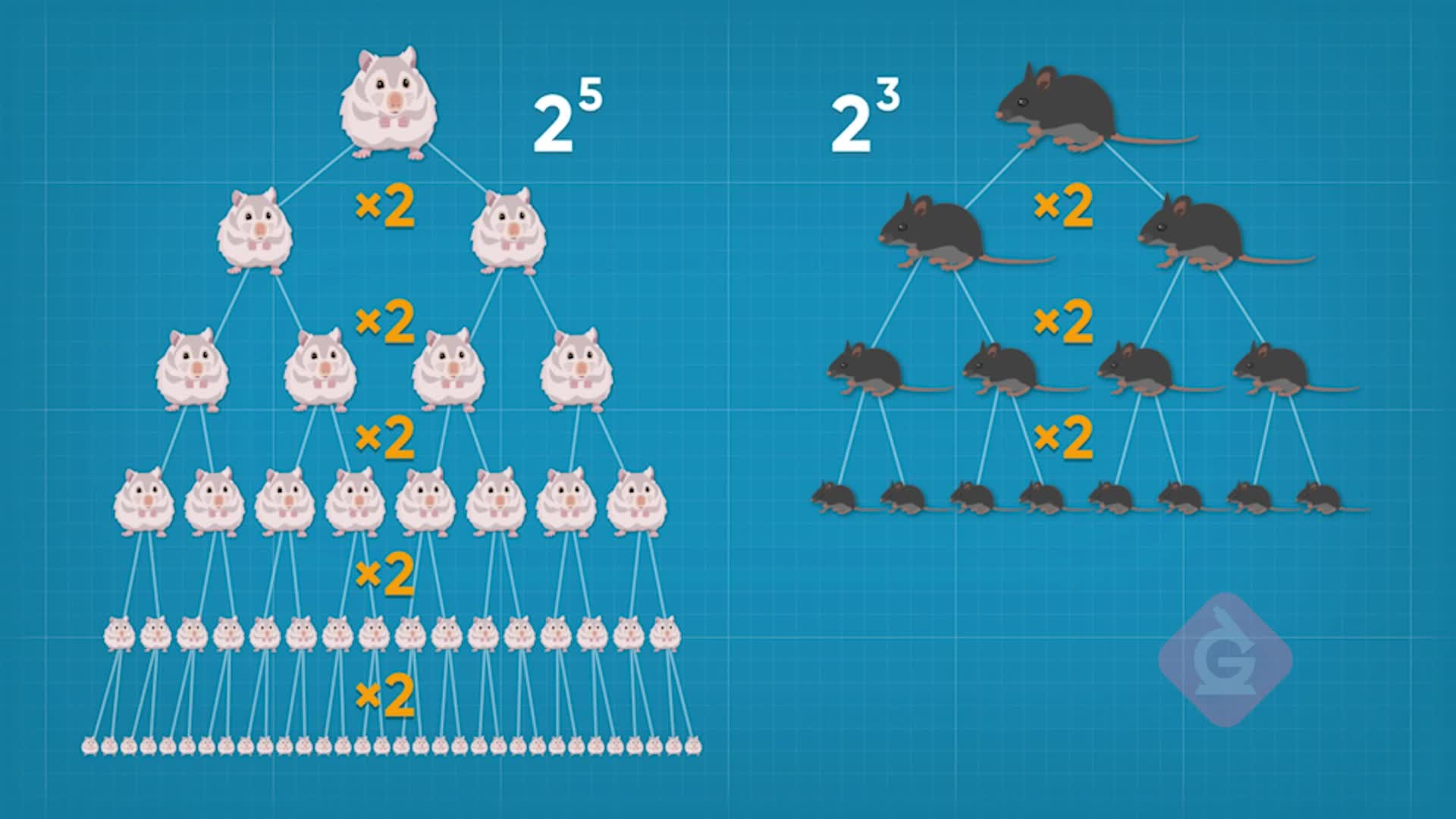WHAT ARE WHOLE NUMBER EXPONENTS?

Students are introduced to exponents and powers. They learn how to derive the exponent laws for multiplying and dividing powers, as well as raising a power to a power.

To better understand whole number exponents…

WHAT ARE WHOLE NUMBER EXPONENTS?. Students are introduced to exponents and powers. They learn how to derive the exponent laws for multiplying and dividing powers, as well as raising a power to a power. To better understand whole number exponents…

## LET’S BREAK IT DOWN!

### Powers of 10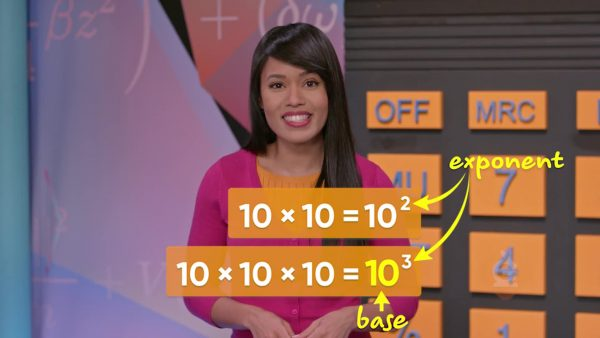If we have 10 × 10, that means we are multiplying by ten two times. We can also express this as 102. 102. We say, “10 to the power of 2.” If we have 10 × 10 × 10 × 10, that’s the same as 104, or “10 to the power of 4.” Now you try: What does 107 mean?

Powers of 10 If we have 10 × 10, that means we are multiplying by ten two times. We can also express this as 102. 102. We say, “10 to the power of 2.” If we have 10 × 10 × 10 × 10, that’s the same as 104, or “10 to the power of 4.” Now you try: What does 107 mean?

### Powers of 2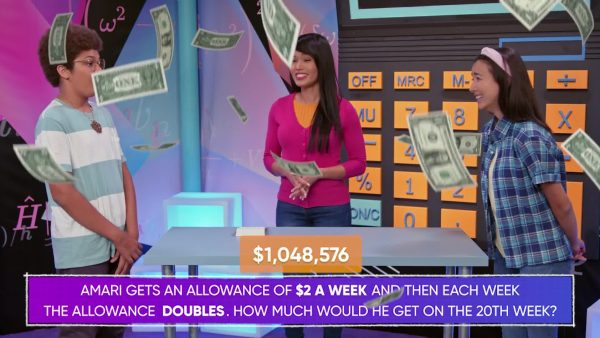You get an allowance that starts at \$2 a week, and each week, your allowance is doubled! How much allowance do you get on the 20th week? This means that we multiplying 2 twenty times. This huge math equation can be made simpler by expressing it using exponents. 2 multiplied 20 times is the same as 220. That’s equal to \$1,048,576! Now you try: How much allowance do you receive on the 11th week?

Powers of 2 You get an allowance that starts at \$2 a week, and each week, your allowance is doubled! How much allowance do you get on the 20th week? This means that we multiplying 2 twenty times. This huge math equation can be made simpler by expressing it using exponents. 2 multiplied 20 times is the same as 220. That’s equal to \$1,048,576! Now you try: How much allowance do you receive on the 11th week?

### Multiplying using exponents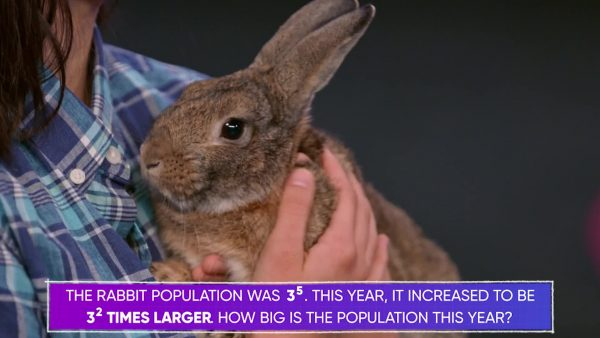The population of rabbits in a town increases rapidly. Last year, the population was 35, and now it is 32 times larger than that! How many rabbits are there now, in all? We can figure this out by multiplying 32 x 35. That is the same as (3×3)×(3×3×3×3×3). Since with multiplication, grouping doesn’t matter, we can rewrite this as 3×3×3×3×3×3×3. That’s 3 multiplied 7 times, which is the same as 37. Notice that 32 x 35 = 37 and 2 + 5 = 7. When we multiply powers that have the same base, we can add the exponents! Now you try: Evaluate 46 x 42.

Multiplying using exponents The population of rabbits in a town increases rapidly. Last year, the population was 35, and now it is 32 times larger than that! How many rabbits are there now, in all? We can figure this out by multiplying 32 x 35. That is the same as (3×3)×(3×3×3×3×3). Since with multiplication, grouping doesn’t matter, we can rewrite this as 3×3×3×3×3×3×3. That’s 3 multiplied 7 times, which is the same as 37. Notice that 32 x 35 = 37 and 2 + 5 = 7. When we multiply powers that have the same base, we can add the exponents! Now you try: Evaluate 46 x 42.

### Powers raised to powers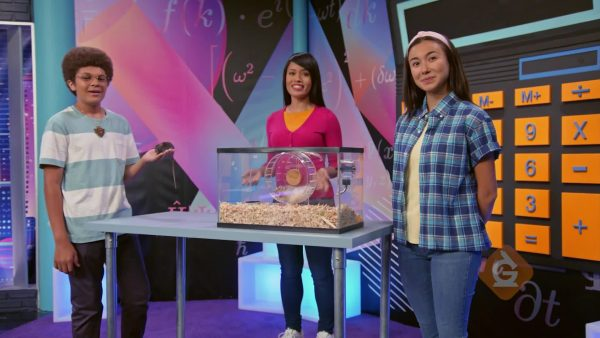At the wildlife sanctuary, the population of mice was 24, and the next year, that amount increased to the third power. As a mathematical expression, that looks like (24)3. This means that 2 is multiplied 4 times, and that product is then multiplied 3 times. To understand how to simplify powers raised to powers, we can expand. 24 means 2 × 2 × 2 × 2, so we can also write (2×2×2×2)3. That means (2×2×2×2)×(2×2×2×2)×(2×2×2×2). If we count, we can see that we multiply 2 a total of 12 times. Notice also that 3 × 4 = 12. Then, (24)3 = 212, which is equal to 4,096. When we raise a power to another power, we multiply the exponents together! Now you try: Evaluate (22)5.

Powers raised to powers At the wildlife sanctuary, the population of mice was 24, and the next year, that amount increased to the third power. As a mathematical expression, that looks like (24)3. This means that 2 is multiplied 4 times, and that product is then multiplied 3 times. To understand how to simplify powers raised to powers, we can expand. 24 means 2 × 2 × 2 × 2, so we can also write (2×2×2×2)3. That means (2×2×2×2)×(2×2×2×2)×(2×2×2×2). If we count, we can see that we multiply 2 a total of 12 times. Notice also that 3 × 4 = 12. Then, (24)3 = 212, which is equal to 4,096. When we raise a power to another power, we multiply the exponents together! Now you try: Evaluate (22)5.

### Dividing using exponents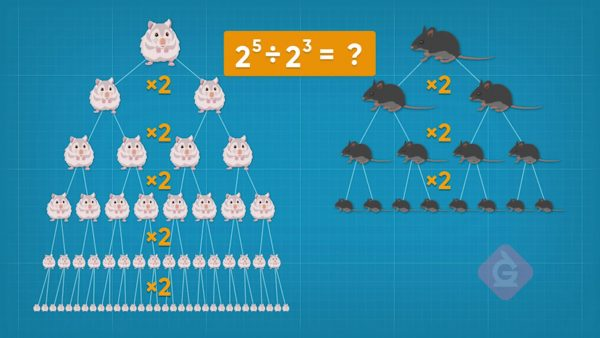A rat family and a hamster family started at the same population. Then the rat family doubled every generation for 3 generations, and the hamster family doubled every generation for 5 generations! How many times larger is the hamster family than the rat family? To solve, we need to represent the hamster and rat populations using exponents, and then divide the hamsters by the rats. Then we have [ggfrac]2⁵/2³[/ggfrac]. We can also express this as [ggfrac]2×2×2×2×2/2×2×2[/ggfrac]. Since any number divided by itself is equal to 1, we can strike out three 2s from the top and three from the bottom. Then all we have left is [ggfrac]2×2/1[/ggfrac], which is equal to 22 = 4. 22=4. The hamster family is 4 times larger than the rat family! When we divide powers that have the same base, we subtract the exponents. Now you try: Evaluate [ggfrac]3⁴/3²[/ggfrac].

Dividing using exponents A rat family and a hamster family started at the same population. Then the rat family doubled every generation for 3 generations, and the hamster family doubled every generation for 5 generations! How many times larger is the hamster family than the rat family? To solve, we need to represent the hamster and rat populations using exponents, and then divide the hamsters by the rats. Then we have [ggfrac]2⁵/2³[/ggfrac]. We can also express this as [ggfrac]2×2×2×2×2/2×2×2[/ggfrac]. Since any number divided by itself is equal to 1, we can strike out three 2s from the top and three from the bottom. Then all we have left is [ggfrac]2×2/1[/ggfrac], which is equal to 22 = 4. 22=4. The hamster family is 4 times larger than the rat family! When we divide powers that have the same base, we subtract the exponents. Now you try: Evaluate [ggfrac]3⁴/3²[/ggfrac].

### Order of operations with exponents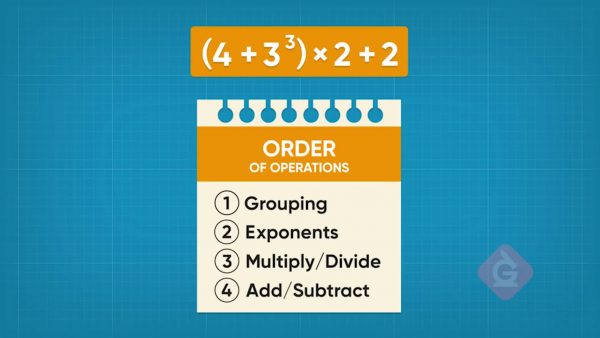GEMS is a way to remember order of operations. It stands for Groupings, Exponents, Multiply and divide left to right, Subtract and add left to right. Evaluate (4 + 33) x 2 + 2 using GEMS. Groupings go first, so find 4 + 33. 33 = 27, so 4 + 33 = 4 + 27 = 31. Now substitute that into the original expression: 31 x 2 + 2. Multiply next. 31 x 2 = 62. Add last. 62 + 2 = 64. So, (4 + 33) x 2 + 2 = 64. Now you try: Evaluate 99 - (3 x 25).

Order of operations with exponents GEMS is a way to remember order of operations. It stands for Groupings, Exponents, Multiply and divide left to right, Subtract and add left to right. Evaluate (4 + 33) x 2 + 2 using GEMS. Groupings go first, so find 4 + 33. 33 = 27, so 4 + 33 = 4 + 27 = 31. Now substitute that into the original expression: 31 x 2 + 2. Multiply next. 31 x 2 = 62. Add last. 62 + 2 = 64. So, (4 + 33) x 2 + 2 = 64. Now you try: Evaluate 99 - (3 x 25).

## WHOLE NUMBER EXPONENTS VOCABULARY

Exponent
Tells how many times a number is multiplied by itself.
Power
A term that uses an exponent.
Base
The number that gets multiplied by itself.
Superscript
A number that is written smaller, a little above and to the right of another number, like an exponent.
GEMS
An acronym that helps us remember the order of operations.
Exponent form
When a term uses exponents, like 25.
When we write a power as a multiplication expression.

## WHOLE NUMBER EXPONENTS DISCUSSION QUESTIONS

### How do you say 25 in words? Which part is called the exponent? Which part is the base?

I say “2 to the power of 5.” It means that 2 is multiplied 5 times. 2 is the base, which is the number I use to multiply. 5 is the exponent, which tells how many times to multiply.

### Does 34 mean the same as 3 × 4?

No. 3 × 4 = 12, but 34 means 3 × 3 × 3 × 3, which is equal to 81. Big difference!

### Adesina needs to evaluate 23 x 25 but she can’t remember the rule for multiplying with powers. How can you help her figure it out?

I can always remember exponent rules by recalling what exponents mean and by expanding the expression, which means writing it the long way. 23 means 2 × 2 × 2 and 25 means 2 × 2 × 2 × 2 × 2. Then 23 x 25 means (2 × 2 × 2) × (2 × 2 × 2 × 2 × 2). I count that 2 is multiplied 8 times, so I can write that as 28. Notice that 3 + 5 = 8, so the rule for multiplying powers with the same base must be that I add together the exponents! I can use my calculator to find out that 28 = 256.

### What is the rule that helps you evaluate (22)4?

When a power is raised to another power, I multiply the exponents. 2 × 4 = 8, so (22)4 = 28 = 256. If I forget the rule, I can expand again!

### Can I use the rule for dividing powers to solve [ggfrac]2⁶/ 3⁶[/ggfrac]?

No. The rule for dividing powers says that if I divide two powers that have the same base, I can subtract their exponents. But 26 and 36 have different bases. That means I can’t use the rule. I can test this answer by expanding and seeing what happens!
X

## Success

We’ve sent you an email with instructions how to reset your password.
Ok

## Choose Your Free Trial Period### 7 DaysContinue to Lessons### 90 DaysGet 90 days free by inviting other teachers to try it too.

Share with Teachers

## Get 90 Days Free

### By inviting 4 other teachers to try it too.

4 required

Skip, I will use a 7 days free trial

## Thank You!

Enjoy your free 90 days trial